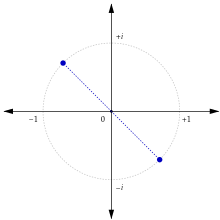"opposite number" redirects here. For other uses, see analog and counterpart.

In mathematics, the additive inverse of a number a is the number that, when added to a, yields zero. This number is also known as the opposite (number), sign change, and negation. For a real number, it reverses its sign: the opposite to a positive number is negative, and the opposite to a negative number is positive. Zero is the additive inverse of itself.

The additive inverse of a is denoted by unary minus: −a (see the discussion below). For example, the additive inverse of 7 is −7, because 7 + (−7) = 0, and the additive inverse of −0.3 is 0.3, because −0.3 + 0.3 = 0 .

The additive inverse is defined as its inverse element under the binary operation of addition (see the discussion below), which allows a broad generalization to mathematical objects other than numbers. As for any inverse operation, double additive inverse has no net effect: −(−x) = x.These complex numbers, two of eight values of 81, are mutually opposite

## Common examples

For a number and, generally, in any ring, the additive inverse can be calculated using multiplication by −1; that is, n = 1 × n . Examples of rings of numbers are integers, rational numbers, real numbers, and complex numbers.

### Relation to subtraction

Additive inverse is closely related to subtraction, which can be viewed as an addition of the opposite:

ab  =  a + (−b).

Conversely, additive inverse can be thought of as subtraction from zero:

a  =  0 − a.

Hence, unary minus sign notation can be seen as a shorthand for subtraction with "0" symbol omitted, although in a correct typography there should be no space after unary "−".

### Other properties

In addition to the identities listed above, negation has the following algebraic properties:

−(a + b) = (−a) + (−b)
a − (−b) = a + b
(−a) × b = a × (−b) = −(a × b)
(−a) × (−b) = a × b
notably, (−a)2 = a2

## Formal definition

The notation + is usually reserved for commutative binary operations, i.e. such that x + y = y + x, for all x, y . If such an operation admits an identity element o (such that x + o ( = o + x ) = x for all x), then this element is unique ( o = o + o = o ). For a given x , if there exists x such that x + x ( = x + x ) = o , then x is called an additive inverse of x.

If + is associative (( x + y ) + z = x + ( y + z ) for all x, y, z), then an additive inverse is unique. To see this, let x and x″ each be additive inverses of x; then

x = x + o = x + (x + x″) = (x + x) + x″ = o + x″ = x″.

For example, since addition of real numbers is associative, each real number has a unique additive inverse.

## Other examples

All the following examples are in fact abelian groups:

• complex numbers: −(a + bi)  =  (−a) + (−b)i. On the complex plane, this operation rotates a complex number 180 degrees around the origin (see the image above).
• addition of real- and complex-valued functions: here, the additive inverse of a function f is the function −f defined by (−f )(x) = − f (x) , for all x, such that f + (−f ) = o , the zero function ( o(x) = 0 for all x ).
• more generally, what precedes applies to all functions with values in an abelian group ('zero' meaning then the identity element of this group):
• sequences, matrices and nets are also special kinds of functions.
• In a vector space the additive inverse v is often called the opposite vector of v; it has the same magnitude as the original and opposite direction. Additive inversion corresponds to scalar multiplication by −1. For Euclidean space, it is point reflection in the origin. Vectors in exactly opposite directions (multiplied to negative numbers) are sometimes referred to as antiparallel.
• In modular arithmetic, the modular additive inverse of x is also defined: it is the number a such that a + x ≡ 0 (mod n). This additive inverse always exists. For example, the inverse of 3 modulo 11 is 8 because it is the solution to 3 + x ≡ 0 (mod 11).

## Non-examples

Natural numbers, cardinal numbers, and ordinal numbers, do not have additive inverses within their respective sets. Thus, for example, we can say that natural numbers do have additive inverses, but because these additive inverses are not themselves natural numbers, the set of natural numbers is not closed under taking additive inverses.